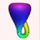Up: The Standard Torus

# Equations for the Standard Torus

The standard torus is paramterized as a surface of revolution: a circle is revolved around an axis. The general equations for such a torus are

```f(u, v) = [ (a + b*cos(v))*cos(u), (a + b*cos(v))*sin(u),
c*sin(v) ]```
The torus shown here was created with
`a = 2, b = 1, c = 1`

source: Alfred Gray, Modern Differential Geometry of Curves and Surfaces.

Up: The Standard TorusThe Geometry Center Home PageTopological Zoo Welcome Page